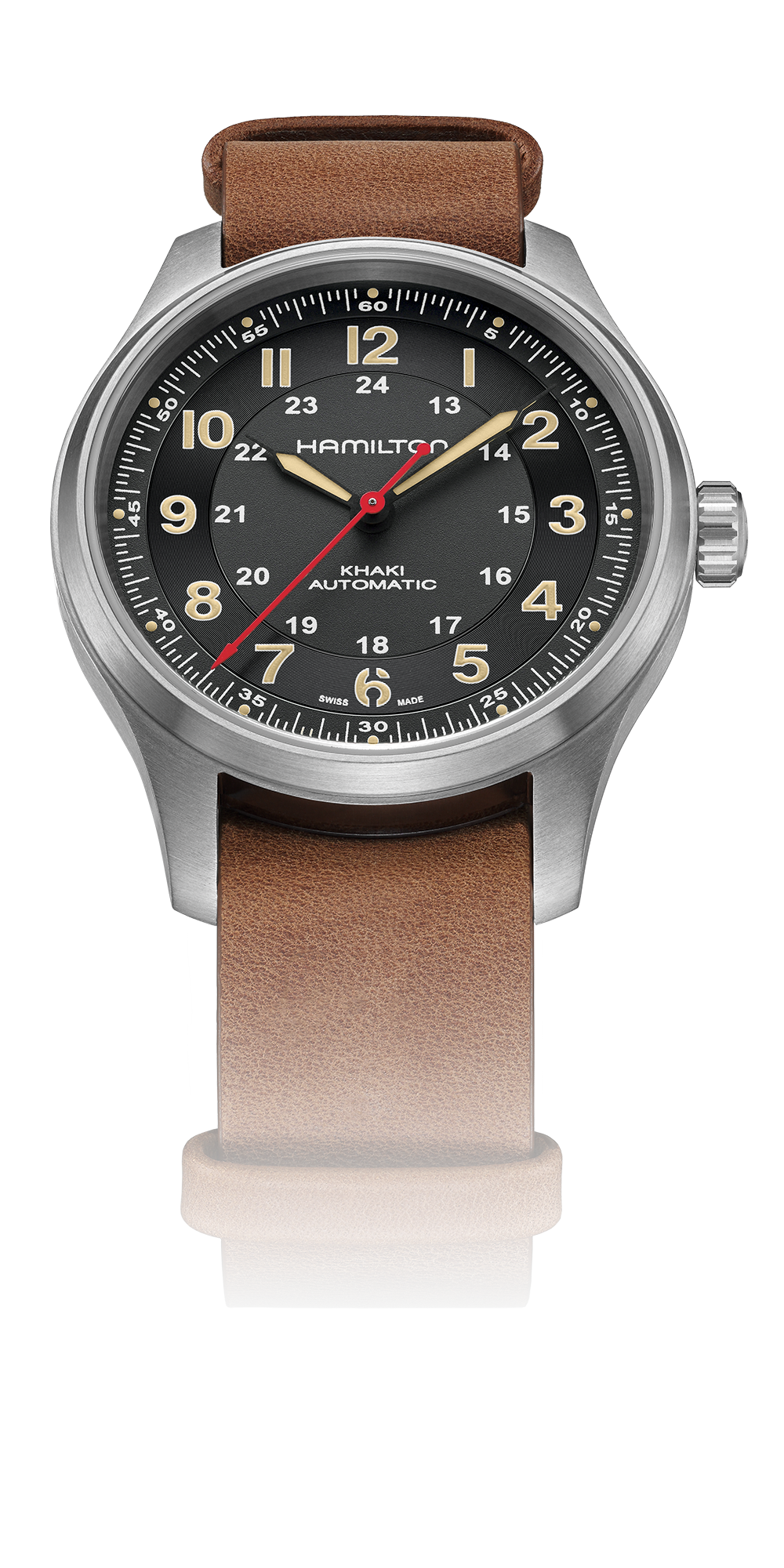# DANI ROJAS

<<<<<<<<<<<<<<<<<<<<<<<<

# 漢米爾頓卡其陸戰系列鈦金屬自動上鍊腕錶《極地戰嚎®6》限量版

<<<<<<<<<<<<<<<<<<<<<<<<